# Nets of solids Online Quiz

Following quiz provides Multiple Choice Questions (MCQs) related to Nets of solids. You will have to read all the given answers and click over the correct answer. If you are not sure about the answer then you can check the answer using Show Answer button. You can use Next Quiz button to check new set of questions in the quiz.Q 1 - Identify the solid whose net is given below.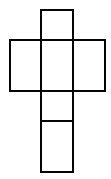### Explanation

Step 1:

If the net is folded along its edges we get a prism.

Step 2:

Since the bases of the prism are congruent rectangles it is the net of a Rectangular prism.

Q 2 - Identify the solid whose net is given below.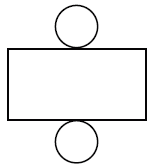### Explanation

Step 1:

If the net is folded along its edges we get a prism.

Step 2:

Since the bases of the prism are congruent circles it is the net of a Cylinder.

Q 3 - Identify the solid whose net is given below.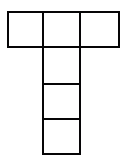### Explanation

Step 1:

If the net is folded along its edges we get a prism.

Step 2:

Since all the faces of the shape are congruent squares, it is the net of a Cube.

Q 4 - Identify the solid whose net is given below.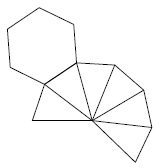### Explanation

Step 1:

If the net is folded along its edges we get a pyramid.

Step 2:

Since the base of the pyramid is a hexagon it is the net of a Hexagonal pyramid.

Q 5 - Identify the solid whose net is given below.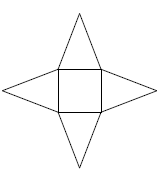### Explanation

Step 1:

If the net is folded along its edges we get a pyramid.

Step 2:

Since the base of the pyramid is a square it is the net of a Square pyramid.

Q 6 - Identify the solid whose net is given below.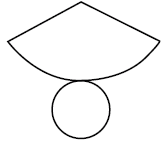### Explanation

Step 1:

If the net is folded along its edges we get a pyramid.

Step 2:

Since the base of the pyramid is a circle it is the net of a Cone.

Q 7 - Identify the solid whose net is given below.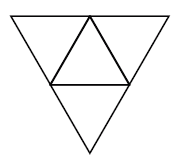### Explanation

Step 1:

If the net is folded along its edges we get a pyramid.

Step 2:

Since the base of the pyramid is a triangle it is the net of a Triangular pyramid.

Q 8 - Identify the solid whose net is given below.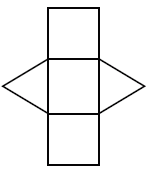### Explanation

Step 1:

If the net is folded along its edges we get a prism.

Step 2:

Since the bases of the prism are congruent triangles it is the net of a Triangular prism.

Q 9 - Identify the solid whose net is given below.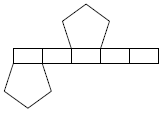### Explanation

Step 1:

If the net is folded along its edges we get a prism.

Step 2:

Since the bases of the prism are congruent pentagons it is the net of a Pentagonal prism.

Q 10 - Identify the solid whose net is given below.### Explanation

Step 1:

If the net is folded along its edges we get a pyramid.

Step 2:

Since the base of the pyramid is a pentagon it is the net of a Pentagonal pyramid.

nets_of_solids.htm